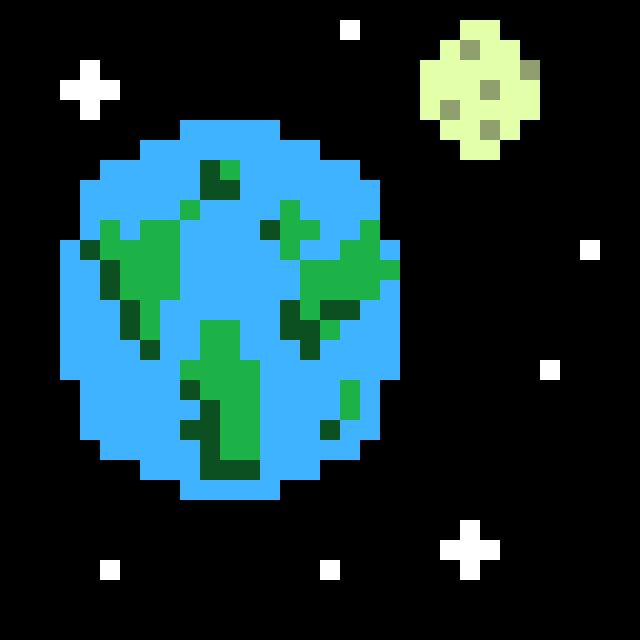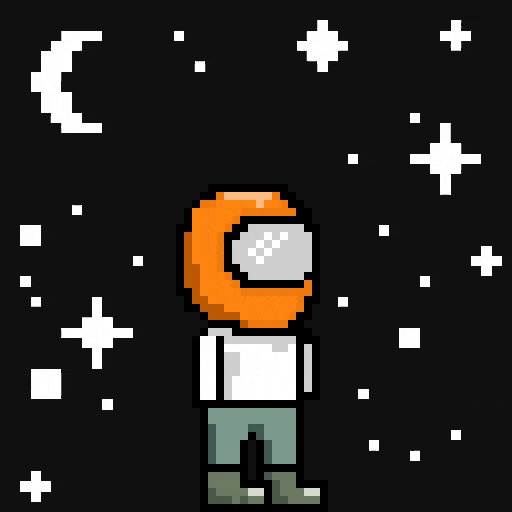# Teach Physics and Programming from the Browser

## p5.js — The intuitive javascript graphics library that just keeps giving.A geographically, physically and artistically incorrect representation of the Earth and Moon — created using Piskel

If you haven’t read my original post about p5.js then I highly recommend you do before starting with this one. It covers all of the basics and will get you up to speed with the p5 library. This post is structured like a lesson with the aim of either teaching people about how to use p5 or showing people how they can captivate their students by making use of p5 in their lessons.

Like I pointed out before, the people at p5 have the humble mission to make:

…coding accessible for artists, designers, educators and beginners…

Originally I was blinded by the potential for artists and graphic designers. I gave little thought to the true potential of the library when concerning educators. Of course, you can show people how graphics work and they can learn through a higher-level abstraction of how real graphics programs are written, but what about other domains? Mathematics? Physics? In this article, we’ll build a physical representation of the Earth and Moon using the true equations that govern their motion, whilst also teaching about programming fundamentals like Object-Oriented Programming, inheritance and methods. If you’re ready, buckle in!

### The Basic Setup

Like all of our p5 sketches we begin with our two fundamental building blocks. The setup() function: this is where we initialise all of the objects and variables we will be using and the draw() function: a loop where we do all of our rendering to the canvas.

``````function setup() {
createCanvas(640, 640);
}

function draw() {
//Updates, renders etc.
}``````

Remember to include the CDNs and your scripts in any HTML document you may be writing this in. If even that’s too much, Codepen is a great sandbox for creating HTML, CSS and javascript masterpieces as well as an environment for teaching.

### The Planet Class

Now that we’ve got the set up out of the way we can start to teach some core programming fundamentals. First of all, what are objects and classes? I like to think of a class as the template or blue-print to building objects. Imagine the blue-prints to a house for example. If I design these well by including things like the functionalities of the house, the types and quantities of materials, then I can go on to build lots of houses based on this theme. That’s a class. All those houses we’re building, they’re the objects.

How does this relate to planets? Well we might want to make lots of planets and we don’t want to have to painstakingly re-write lines of code to construct new planets everytime! So, we define a blue-print. A class called Planet.

When coding classes it’s always a good thing to think “what are the bare minimum things I need to define what I’m building?”. For a planet it could be a position, velocity, mass and radius. And if we’re being artists, a colour as well. And what functionality should it have? Well for now, let’s just draw it.

``````class Planet {
//The 'build' method
constructor(position, mass, radius, color) {
this.position = position;
//A p5 Vector
this.velocity = createVector(0, 0);
this.mass = mass;
this.radius = radius;
this.color = color;
}

show() {
//pushing and popping is like save and restore
push();
//that way we can translate over to here
translate(this.position.x, this.position.y);
fill(this.color);
//draw our planet
ellipse(0, 0, this.radius, this.radius);
//and pop back to wherever we were before
pop();
}
}``````

### The Satellite Class

So now we want to have a Moon too. The Moon is a natural satellite and in the future we might want more satellites (like the International Space Station), so we’re better off generalising our next class to a Satellite class.

One thing to always remember is, for the most part, programmers are lazy. Rewriting code takes time and not only that, but it allows bugs to creep into your code like reassigning a variable or changing arrays you didn’t want to. The astute amongst you may have realised that a Satellite, like the Moon, is an awful lot like a planet. In fact, if we could just inherit all of the characteristics of the Planet class and then just define the add-ons that a Satellite has that would save us time and lines of code. And we can!

Inheritance is the ability for a class to become a subclass of another superclass. In order to achieve this we use the extends keyword in javascript along with a special call to a super() function. This just tells the superclass to do the same constructing it would normally do. So, what else should our Satellite do? Well it’s going to be moving so we need an update() function, and there will also be a force applied to it so an applyForce(force) function also makes a lot of sense. One thing we didn’t define before was an acceleration, so we can do that now too!

``````class Satellite extends Planet {
constructor(position, mass, radius, color, vel) {
//Telling Planet to build a base planet for us
super(position, mass, radius, color);
//Redefining the velocity to be something we pass it (shadowing)
this.velocity = vel;
//Our new variable acceleration
this.acceleration = createVector(0, 0);
}

//An apply force function - check the physics section to understand this
applyForce(force) {
var f = p5.Vector.div(force, this.mass);
this.acceleration.add(f);
}

//And an update function
update() {
this.velocity.add(this.acceleration)
this.position.add(this.velocity);
this.acceleration.mult(0);
}
}``````

### The Physics

We’ve already introduced some physics when we built our satellite class, one of the most widely taught and beautiful equations. Newton’s second law:

force = mass x acceleration

In our case we were applying an external force to our satellite and we already knew the mass so what we really wanted to change was the acceleration. Lucky for us the p5 library makes vector maths incredibly simple. Using vectors to represent position, velocity and acceleration is incredibly useful in teaching. Often people just assume these values to be scalars, but they’re not! Using this approach to teaching them makes this abundantly clear. Our function then looks like this.

``````//applying a force to our object (force is also a vector)
applyForce(force) {
// f = ma -> f/m = a
let f = p5.Vector.div(force, this.mass);
this.acceleration.add(f);
}``````

Another common stumbling block for students is the relationship between position, velocity and acceleration. Telling students that velocity is the change in position with respect to time is a little obtuse, especially if they’re new to the subject. In our update() function, however, it becomes clear how they relate. The acceleration nudges the velocity which in turn nudges the position before resetting the acceleration to zero. Why? Just ask Isaac Newton! Unless more force is being applied to our object we don’t want the acceleration to constantly increase otherwise our object would indefinitely speed up.

So far so good. But, what force are we actually applying here? What force keeps the Moon orbiting the Earth? The centripetal force. The equation for calculating the force is f = (m * v^2)/r where m is the mass of the Moon, v is the Moon’s velocity and r is the distance to the centre of the Earth. One more important point is the direction of the force. The centripetal force is always directed towards the centre of rotation. We can calculate this direction quite easily using the position vectors of the Earth and the Moon:

let force = p5.Vector.sub(satellite.position, centre.position).normalize();

Notice we have to normalize() this force because we only want its direction not any associated magnitude, that comes from the equation above. Our full function looks something like this.

``````function centripetalForce(satellite, centre) {
//Get the direction of the force
let force = p5.Vector.sub(centre, satellite.position).normalize();
//Get the distance to centre of rotation from our satellite
let radius = p5.Vector.sub(centre, satellite.position).mag();
//The velocity squared
let velocitySquared = p5.Vector.dot(satellite.velocity, satellite.velocity);
//Pull it all together with the f = (m * v^2) / r equation
force.mult(velocitySquared);
force.mult(satellite.mass);
force.mult(1/radius);
return force;
}``````

Great! Now we have all the pieces to put our Earth-Moon model together.

### The Model

Let’s create our Earth and Moon as variables in the global scope and then set them in our setup() function. This is common practice in a lot of programming and in fact is often the cause of a lot of headaches when you forget to initialise your variables and wonder why you have null pointer exceptions everywhere.

``````//Our global variables
let earth;
let moon;
let r = 225;

function setup() {
createCanvas(640, 640);
//Creating our Earth and Moon
earth = new Planet(createVector(width/2, height/2), 1000, 100, 'blue');
//Notice we're starting the moon directly 'east' to our Earth, so the initial velocity is straight upwards.
//Feel free to experiment with this initial velocity to see how it affects the orbit
moon = new Satellite(createVector(width/2 + r, height/2), 10, 50, 'white', createVector(0, -4));
}

function draw() {
//Clear the canvas
background(0);
//Draw the Earth
earth.show();
//If the Moon is close enough to the Earth, apply the force
if(getDistance(earth, moon) <= r) {
moon.applyForce(centripetalForce(moon, earth.position));
}

//Update the moon
moon.update();
//Render it to the canvas... and then loop back to the start
moon.show();
}``````

And that’s it! We now have a fully functioning Earth and Moon model. We’ve been able to teach some of the core programming fundamentals, as well as offering a more intuitive and visual understanding of the laws of motion. All in our browser of choice! For a full demo check out the Codepen below.

I hope this article can inspire you to create your own educative experiences in the browser using p5.js. This has only scratched the surface. Two great resources to inspire you even more are:

Daniel Shiffman’s The Coding Train who I owe a lot of how I got interested in programming too.

The p5 examples page and in particular the “simulate” subsection.

Happy coding and creating!